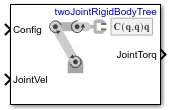Velocity Product Torque

Joint torques that cancel velocity-induced forces

• Library:
• Robotics System Toolbox / Manipulator Algorithms

•Description

The Velocity Product Torque block returns the torques that cancel the velocity-induced forces for the given robot configuration (joint positions) and joint velocities for the Rigid body tree robot model.

Ports

Input

expand all

Robot configuration, specified as a vector of positions for all nonfixed joints in the robot model, as set by the Rigid body tree parameter. You can also generate this vector for a complex robot using the homeConfiguration or randomConfiguration functions inside a Constant or MATLAB Function block.

Joint velocities, specified as a vector. The number of joint velocities is equal to the degrees of freedom (number of nonfixed joints) of the robot.

Output

expand all

Joint torques, specified as a vector. Each element corresponds to a torque applied to a specific joint. The number of joint torques is equal to the degrees of freedom (number of nonfixed joints) of the robot.

Parameters

expand all

Robot model, specified as a rigidBodyTree object. You can also import a robot model from an URDF (Unified Robot Description Formation) file using importrobot.

The default robot model, twoJointRigidBodyTree, is a robot with revolute joints and two degrees of freedom.

• Interpreted execution — Simulate model using the MATLAB® interpreter. This option shortens startup time but has a slower simulation speed than Code generation. In this mode, you can debug the source code of the block.

• Code generation — Simulate model using generated C code. The first time you run a simulation, Simulink® generates C code for the block. The C code is reused for subsequent simulations, as long as the model does not change. This option requires additional startup time, but the speed of the subsequent simulations is comparable to Interpreted execution.

Tunable: No

Extended Capabilities

C/C++ Code GenerationGenerate C and C++ code using Simulink® Coder™.

Introduced in R2018a